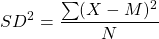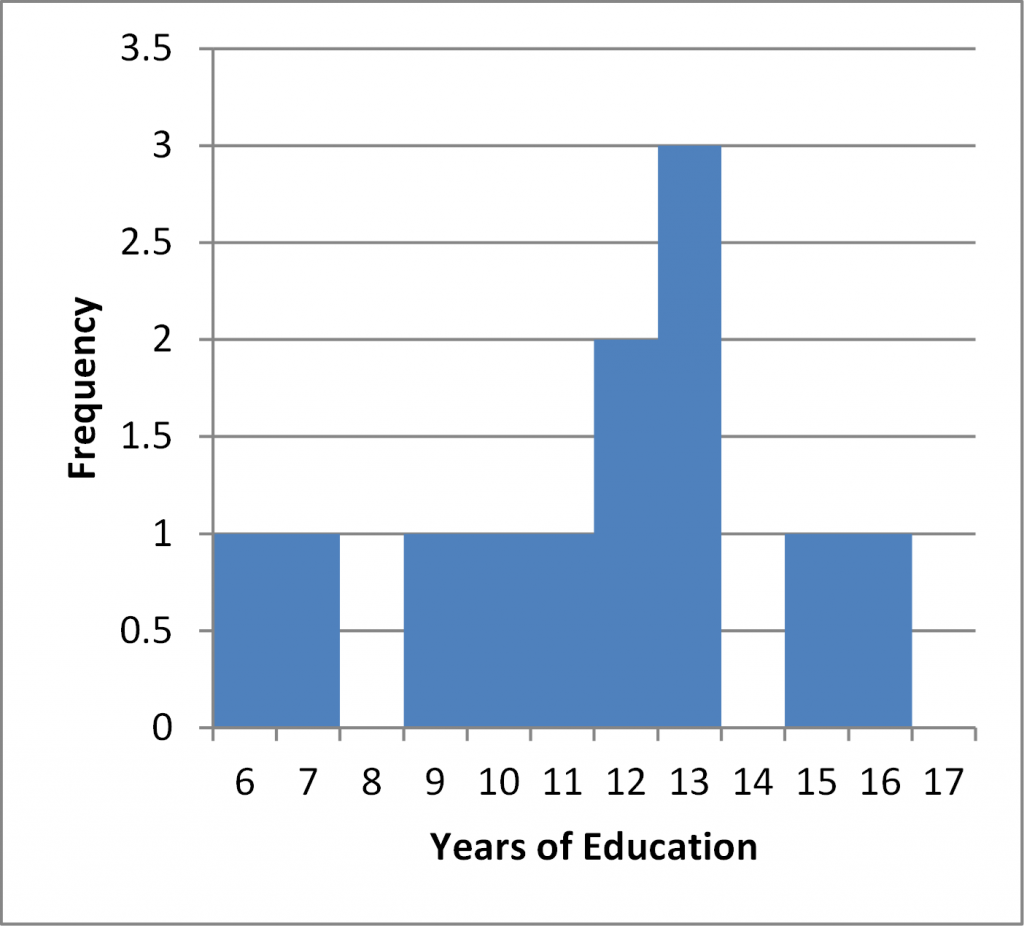# Worksheet 2b.

These guided exercises will give you the opportunity to practice the techniques and apply the concepts you learned in Chapter 2b. You will need some paper, spreadsheet, or a tablet to work on the analyses.

1. Data collected from hospital records reveal that the age of first hospitalization for psychosis for a sample of patients are:

18, 20, 21, 22, 23, 23, 24, 28, 28, 29, 32, 37, 39

a. Calculate the variance for this dataset using the formula below.1. Steps to Calculate Variance

1. Take the distance (“deviation”) of each score from the mean
2. Square each distance to get rid of the sign (because some deviations will be negative)
3. Add up all the resulting “squared deviations” to get Sum of Squares (SS)
4. Divide by the number of scores

b. Calculate the standard deviation for this dataset using the formula below.1. The number of years of education for most members of the sample in the study of psychosis onset were:

6, 7, 9, 10, 11, 12, 12, 13, 13, 13, 15, 16

a. Calculate the variance for this dataset using the formula below.1. b. Calculate the standard deviation for this dataset using the formula below.